## Matrix Subtraction

When we have two matrices,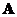and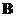then the matrix subtraction or matrix difference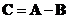is a matrix whose entry is formed by subtracting the corresponding entry offrom each entry of, that is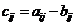.

Matrix subtraction works only when the two matrices have the same size and the result is also the same size as the input matrices.

Example,,The interactive program below shows you the result of matrix subtraction. Your input must be two matrices of the same size (one matrix for each text box). Random Example will generate random matrices of the same size.

-

## Properties

Some important properties of matrix difference are

• Matrix subtraction is a non-commutative operation. If you reverse the order you will not get the same result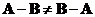• Null matrix (or zero matrix) is a unique difference identity matrix such that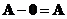. The null matrix has the same size as matrix.
• We can define a negative of(written as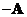) such that matrix addition with the negative will produce a null matrix ,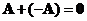See also : matrix addition , null matrix